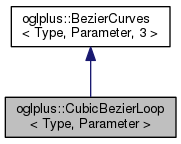OGLplus (0.52.0) a C++ wrapper for OpenGLoglplus::CubicBezierLoop< Type, Parameter > Class Template Reference

A closed smooth cubic Bezier spline passing through all input points. More...

`#include </home/chochlik/devel/oglplus/include/oglplus/math/curve.hpp>`

Inheritance diagram for oglplus::CubicBezierLoop< Type, Parameter >:[legend]
Collaboration diagram for oglplus::CubicBezierLoop< Type, Parameter >:[legend]

## Public Member Functions

CubicBezierLoop (const ::std::vector< Type > &points, Parameter r=Parameter(1)/Parameter(3))
Creates a loop passing through the sequence of the input points.

bool Connected (void) const
Returns true if the individual curves are connected.

bool Separated (void) const
Returns true if the individual curves are connected.

unsigned SegmentCount (void) const
Returns the count of individual curves in the sequence.

const ::std::vector< Type > & ControlPoints (void) const
Returns the contol points of the curve.

Type Position01 (Parameter t) const
Gets the point on the curve at position t (must be between 0.0, 1.0)

Type Position (Parameter t) const
Gets the point on the curve at position t wrapped to [0.0, 1.0].

void Approximate (std::vector< Type > &dest, unsigned n) const
Makes a sequence of points on the curve (n points per segment)

::std::vector< Type > Approximate (unsigned n) const
Returns a sequence of points on the curve (n points per segment)

BezierCurves< Type, Parameter,
Order-1 >
Derivative (void) const
Returns a derivative of this curve.

## Static Public Member Functions

static bool PointsOk (const ::std::vector< Type > &points)
Checks if the sequence of control points is OK for this curve type.

static Parameter Wrap (Parameter t)
Wraps the parameter value to [0.0, 1.0].

## Detailed Description

### template<typename Type, typename Parameter> class oglplus::CubicBezierLoop< Type, Parameter >

A closed smooth cubic Bezier spline passing through all input points.

This class constructs a closed sequence of Bezier curves that are smooth at the curve connection points. The control points between the begin and end points of each segment are calculated automatically to make the transition between the individual segments smooth.

The documentation for this class was generated from the following file:
• /home/chochlik/devel/oglplus/include/oglplus/math/curve.hpp